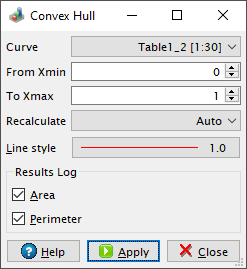# Convex Hull Dialog

This dialog is activated by selecting the Convex Hull... command from the Analysis menu.

In geometry, the convex hull of a set of points in the plane is the smallest convex polygon that contains all the points of that set. In order to detect the convex hull, QtiPlot uses Graham scan algorithm.

Figure 5-129. The Convex Hull DialogBy default, a log message displaying the area, the perimeter and the number of points of the convex hull, is written to the results log panel. For the calculation of the area of the convex hull, QtiPlot uses the shoelace formula. It is possible to disable the calculation of these values by unchecking the Area and Perimeter boxes from the Results Log group box.